# Multiple choice questions on linear momentum and system of particles for JEE/AP physics

In this page we have Objective type questions on linear momentum and system of particles for CBSE,JEE Advanced/Main, AP Physics . Hope you like them and do not forget to like , social share and comment at the end of the page.
Multiple choice Questions
Question 1. A 5kg rifle fires a 6g bullet with speed of 500m/s. Find the ratio of the distance the rifle move backward while the bullet is in the barrel to the distance the bullet moves forward?
(a) 1/600
(b) 1/666
(c) 2/327
(d) none of the above
Question  2. A neutron of mass m is collides elastically with a nucleus of mass M, which is initially at rest. Neutron kinetic energy is k0. Find the maximum kinetic energy it can lose
(a)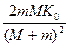(b)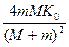(c)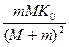(d) none of the above

Question 3. A grenade of mass M is falling with a velocity v0 at height when it explodes into two equal fragments that initially move horizontally in center of mass frame. The explosion is such that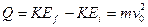Determine where the fragment will fall on the ground relative to the point directly below the grenade at the time of explosion
(a)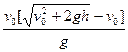(b)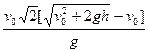(c)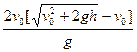(d) None of the above

A projectile (mass m) is fired from Origin O with an initial velocity of u at angle θ above the horizontal in the X-Y plane
Question 4 Find the x, y and z component of the angular momentum of the projectile about point O as a function of time
(a)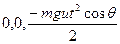(b)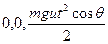(c)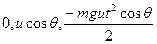(d)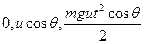Question 5 Calculate the torque of the weight acting on the projectile about the Origin
a)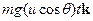b)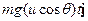c)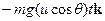d) None of the above
Multiple Choice Questions
Question 6
Position vector of a body of mass 6 Kg is given as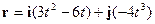Find the torque acting on it about the origin
a)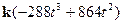b)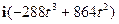c)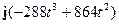d) None of these
A system is composed of two particles having mass m1 and m2 respectively. They are connected by a light spring of spring constant K. At time t=0, the system is thrown in the air such that m1 has initial velocity v1   and m2 has initial velocity v2 . Neglect any air drag in the motion
Question 7 Find the total momentum of the system at time t
a)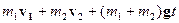b)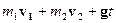c)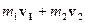d) None of these
Question 8 Find the radius vector of the center of the mass relative to initial position.
a)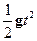b)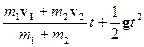c)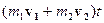d) None of these
A person Jack stands at left end of a railway car sitting on  a railway track. There is no frictional force between the track and the railway car.
Person + Railway track Mass =M
Length of the railway car         =L
Jack throws a ball of mass m with velocity u towards the right end of the railway car where it collides elastically with the wall and travel back to the other end and strike the wall inelastically  and coming to rest.
i is the unit vector across right end.
Question 9 Match the column
Column A
P) Velocity of the railway car after the first collision
Q) Velocity of the railway car after the second collision
R) Velocity of the railway car before first collision
S) Velocity of the Center of the mass of system( Railway car + jack + ball)
Column B
A) 0
B)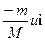C)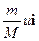D)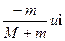Question 10  Which of the following statement is true
a) The ball will take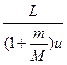time to strike the right end
b) The ball will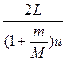time to strike the left end
c) There will be no net displacement of the railway car at the end of timed) There will no change in center of the mass position of the system (Railway car + jack + ball)
After falling from rest through a distance h, a body of mass m begin to raise a body of Mass M (M >m) that is connected to it by means of a light inextensible string passing over a fixed smooth pulley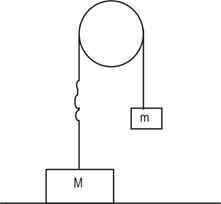Question 11 Find the velocity of the mass after the jerk
a)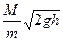b)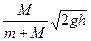c)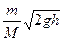d)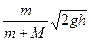Question  12 Find the time taken for the body of mass M to return to its original position
a)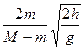b)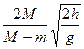c)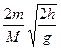d) None of these
Question 13 Find the fraction of the KE lost after the first Jerk
a)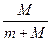b)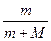c)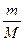d) None of these
Multiple choice Questions
Question 1. A 5kg rifle fires a 6g bullet with speed of 500m/s. Find the ratio of the distance the rifle move backward while the bullet is in the barrel to the distance the bullet moves forward?
(a) 1/600
(b) 1/666
(c) 2/327
(d) none of the above
Question  2. A neutron of mass m is collides elastically with a nucleus of mass M, which is initially at rest. Neutron kinetic energy is k0. Find the maximum kinetic energy it can lose
(a)(b)(c)(d) none of the above

Question 3. A grenade of mass M is falling with a velocity v0 at height when it explodes into two equal fragments that initially move horizontally in center of mass frame. The explosion is such thatDetermine where the fragment will fall on the ground relative to the point directly below the grenade at the time of explosion
(a)(b)(c)(d) None of the above

A projectile (mass m) is fired from Origin O with an initial velocity of u at angle θ above the horizontal in the X-Y plane
Question 4 Find the x, y and z component of the angular momentum of the projectile about point O as a function of time
(a)(b)(c)(d)Question 5 Calculate the torque of the weight acting on the projectile about the Origin
a)b)c)d) None of the above
Multiple Choice Questions
Question 6
Position vector of a body of mass 6 Kg is given asFind the torque acting on it about the origin
a)b)c)d) None of these
A system is composed of two particles having mass m1 and m2 respectively. They are connected by a light spring of spring constant K. At time t=0, the system is thrown in the air such that m1 has initial velocity v1   and m2 has initial velocity v2 . Neglect any air drag in the motion
Question 7 Find the total momentum of the system at time t
a)b)c)d) None of these
Question 8 Find the radius vector of the center of the mass relative to initial position.
a)b)c)d) None of these
A person Jack stands at left end of a railway car sitting on  a railway track. There is no frictional force between the track and the railway car.
Person + Railway track Mass =M
Length of the railway car         =L
Jack throws a ball of mass m with velocity u towards the right end of the railway car where it collides elastically with the wall and travel back to the other end and strike the wall inelastically  and coming to rest.
i is the unit vector across right end.
Question 9 Match the column
Column A
P) Velocity of the railway car after the first collision
Q) Velocity of the railway car after the second collision
R) Velocity of the railway car before first collision
S) Velocity of the Center of the mass of system( Railway car + jack + ball)
Column B
A) 0
B)C)D)Question 10  Which of the following statement is true
a) The ball will taketime to strike the right end
b) The ball willtime to strike the left end
c) There will be no net displacement of the railway car at the end of timed) There will no change in center of the mass position of the system (Railway car + jack + ball)
After falling from rest through a distance h, a body of mass m begin to raise a body of Mass M (M >m) that is connected to it by means of a light inextensible string passing over a fixed smooth pulleyQuestion 11 Find the velocity of the mass after the jerk
a)b)c)d)Question  12 Find the time taken for the body of mass M to return to its original position
a)b)c)d) None of these
Question 13 Find the fraction of the KE lost after the first Jerk
a)b)c)d) None of these

Solutions